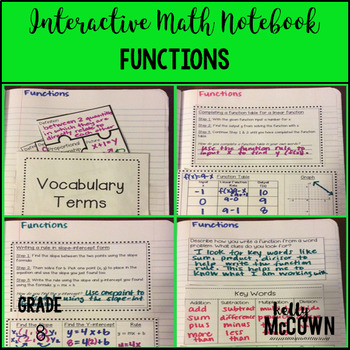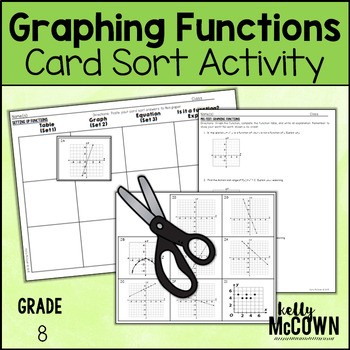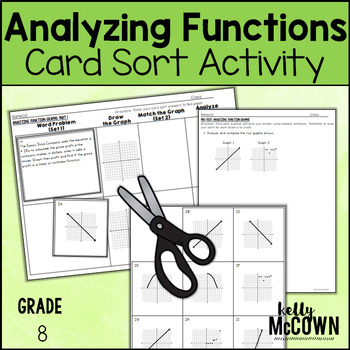## Wednesday, November 9, 2016

A fun Pre-Algebra topic to teach in eighth grade math is functions. Students have prior knowledge of numbers, expressions, and variables which helps them to be able to write and find functions.

I have listed here five activities to review and practice Functions. I hope these help jump start your lesson planning into Functions. The key to getting your students involved in the learning process is to include interactive, visual, engaging activities for them to actively participate in.These interactive notebook activities are intended to help students understand how to graph proportional relationships, understand that a function is a rule that assigns one input to one output exactly, compare properties of two functions, interpret the equation y = mx + b, construct a function, determine the rate of change of a function, and describe the functional relationship between two quantities.

Included are:
-4 different Functions activities to engage students
-Teacher facilitated activity for 60-90 minutes of classroom time
-Activities include: Vocabulary, Completing a function table and graph from a linear function rule, Writing a rule from two points using slope-intercept form, and Writing a rule, completing a function table, and drawing a graph from a function word problem.
-Examples of Completed Interactive Math Notebook Activities are includedThis math station activity is intended to help students understand how to graph proportional relationships, understand that a function is a rule that assigns one input to one output exactly, compare properties of two functions, interpret the equation y = mx + b, construct a function, determine the rate of change of a function, and describe the functional relationship between two quantities.

Included are:
-6 different stations to engage students
-Teacher facilitated activity for 60-90 minutes of classroom time
-Stations include:
1. Vocabulary
3. Practice of Functions
5. Real World applications of Functions
6. Word problems of Functions
-Student Station Guide helps students record their answersThis lesson unit is intended to help you assess how well students are able to assess and graph functions in tables, graphs, and equations.Included in this lesson are:
-card sort activity with concept development of functions
-one extension activity for students to deepen their conception of functions
-answer keys for all the assessments and activities
-how the lesson is tied to common core and mathematical practicesThis lesson unit is intended to help you assess how well students are able to describe qualitatively the functional relationship between two quantities by analyzing a graph (e.g., where the function is increasing or decreasing, linear or nonlinear). Sketch a graph that exhibits the qualitative features of a function that has been described verbally.Included in this lesson are:
-card sort activity with concept development of analyzing functions
-one extension activity for students to deepen their conception of analyzing functions
-answer keys for all the assessments and activities
-how the lesson is tied to common core and mathematical practicesThis November Math NO PREP packet that will keep your eighth graders engaged! This packet is just plain fun. Not only is it PACKED with eighth-grade common core math problems, it also gives students fun coloring, puzzles, and problem solving. Use this packet for bellwork, classwork, extra credit, fast finishers, or homework!
Topics Covered:
-Operations with Exponents
-Proportional Relationships
-Scientific Notation
-Graphing
-Equations
CLICK HERE to watch the video tutorial of the NO PREP Math Packets for November

Have fun with Functions! Find a creative approach and teach it. November is a short and hard time to engage your students. YET IT IS the perfect time to engage your students in Math and the rewards will be higher in the end of year!